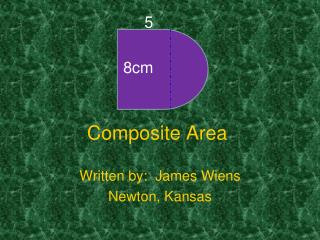DownloadDownload PresentationComposite Area

# Composite Area

Télécharger la présentation## Composite Area

- - - - - - - - - - - - - - - - - - - - - - - - - - - E N D - - - - - - - - - - - - - - - - - - - - - - - - - - -
##### Presentation Transcript

1. 5 8cm Composite Area Written by: James Wiens Newton, Kansas

2. Instructor Notes • Subject Area(s): Math • Grade level: 7th grade • Lesson Length: 50 minute class period • Synopsis: Solve for area of composite figures • Objective/goals: Students will find the area of composite figures by breaking them into common shapes with formulas such as triangles, quadrilaterals and circles.

3. Instructor Notes • Standard: • c. Kansas standard 7.3.2.A1c▲ ■ finding perimeter and area of two-dimensional composite figures of squares, rectangles, and triangles (2.4.A1h), e.g., the front of a barn is rectangular in shape with a height of 10 feet and a width of 48 feet. Above the rectangle is a triangle that is 7 feet high with sides 25 feet long. What is the area of the front of the barn? • Pre-requisite skills: Vocabulary – Area, base, height, triangle, paralellogram, trapezoid, pi, radius, circle. • TurningPoint functions:standard question slides • Materials: All instructional points and practice problems are provided within the power point slides. Practice questions are designed to be used with the TurningPoint clickers.

4. Lesson Outline • Warm-up: find area of basic shapes • Definitions / Key Concepts • Setting the Stage: Video lesson • Guided practice: Turning Point Questions • Independent practice: Paper & pencil • Closure: Write about area

5. Answer Now Find the area. • 19 in2 • 44 in2 • 88 in2 8in 11in 10 Countdown

6. Answer • Area parallelogram = base x height • A = bh • A = 8 (11) • A = 88 in.2

7. Answer Now Find the area. • 30 in2 • 60 in2 • 120 in2 6in 10in 10 Countdown

8. Answer • Area triangle = ½ (base x height) • A = ½ bh • A = ½ (6)(10) • A = ½ (60) • A = 30 in.2

9. Answer Now Find the area. (Use 3.14 for π) • 62.8 in2 • 314 in2 • 31.4 in2 10in 10 Countdown

10. Answer • Area circle = π r2 • A = π r2 • A = π (10)2 • A = π (100) • A = 314 in.2

11. Definition • Area of complex figures • To find the area of a complex figure, break the shape into two or more simple figures. Find the area for the simple figures then combine those amounts to find the total of the complex figure.

12. How much area does the blue figure cover? Setting the Stage 4 3 9 4

13. Answer • A = bh • A= 4 (9) • A = 36 in.2 • A = ½ bh • A = ½ (4)(3) • A = ½ (12) • A = 6 in.2 Final Answer: 36 + 6 = 42 in.2

14. Video Clip Lesson from Teacher Tube • Click on the link at the right to access a lesson about area of composite figures from Holt Mathematics. Click here to see the lesson

15. Answer Now What is the area of this figure? • 36 cm2 • 54 cm2 • 45 cm2 • 18 cm2 3 cm 9 12 3 3 6 10 Countdown

16. Answer Now What is the area of this figure rounded to the nearest cm2?(Use 3.14 for π) • 40 cm2 • 90 cm2 • 126 cm2 • 241 cm2 5 8cm 10 Countdown

17. Answer Now What is the area of this figure? • 114 cm2 • 108 cm2 • 87 cm2 • 72 cm2 5 12 2 2 6 8 10 Countdown

18. Independent Practice - Find the area of each figure rounded to the nearest foot (All units in feet ). A. C. 50 10 6 30 30 14 50 B. D. 7 6 10 5 5 4 15 12 4

19. Answer Key for Independent Practice

20. Closure / Summary • Explain how finding the area of a right angle trapezoid is the same as using the sum of the areas of a triangle and a rectangle.

21. References • Video clip from slide # 14 found at http://my.hrw.com/math06_07/nsmedia/lesson_videos/msm1/player.html?contentSrc=6072/6072.xml (Holt McDougal math series, Houghton Mifflin Harcourt.) • Remainder of lesson designed and written by James Wiens, 7th grade math teacher, Newton Kansas.